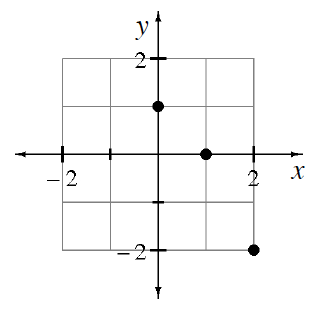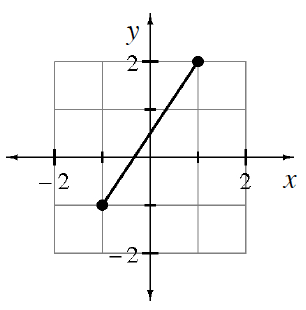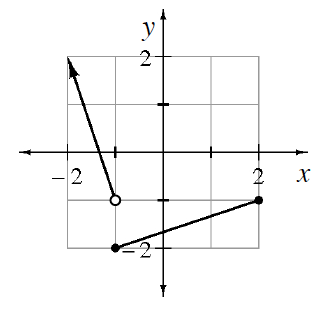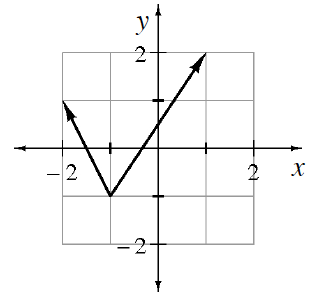### Home > INT1 > Chapter 7 > Lesson 7.1.2 > Problem7-24

7-24.

State the domain and range for each graph below.

Read Math Notes box for lesson 1.2.5 on functions, domains and ranges.

1.Domain: ${0, 1, 2}$
Range: ${-2, 0, 1}$

1.Domain: $-1 ≤ x ≤ 1$
Range: $-1 ≤ y ≤ 2$

1.Domain: $x ≤ 2$
Range: $y ≥ −2$

1.Domain: all real numbers
Range: $y ≥ -1$